copyright 1998-2018 by Mark Verboom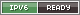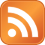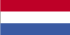Go back to What's new

Engine power measurement

Saturday, 16 April, 2011

Engine power measurement

Today we did a power measurement of this car:A Golf VI 2.0 GTD.

The basic idea behind the engine power measurement is to measure the acceleration on a fixed mass. If you know those two variables, you're able to determine the amount of energy that was required. Using some more math you can make your own dynoplots that way :)

To start of we determined the mass of the car. To do this we setup my compuscales and rolled the car on.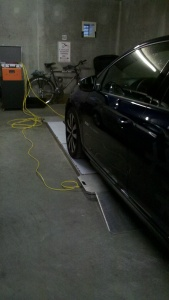The it is just a matter of reading the data on the display.Now that we know the mass, we have to determine the acceleration. To keep it mostly legal and lower the aerodynamic drag influence, the test is done in 3rd gear. But before accelerating the car, we put the data logger in place.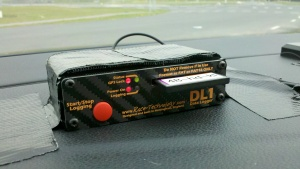This is a Race Technology DL1 datalogger. It has 3 access accelerometer, gyro sensor, 5Hz GPS and lots of extra inputs. All data can be logged 100 times per second.

While running the car in 3rd gear we did a couple of pulls from 1000 rpm to the red line. That gives us the acceleration information.

But for a dynoplot you also need the RPM of the engine. Because this was just a quick install, we didn't make an RPM pickup from somewhere behind the dash. To still get the RPM, we did a couple of test by driving the car in 3rd gear at fixed RPM and read the speed of the car computer. That gives you the ratio of the engine RPM versus the speed of the car.

Using the Race Technology free Analysis software, we loaded the data and entered some basic information on the car:

• weight
• roling resistance
• aerodynamic drag
The last two can be determined by running a few coastdown tests at different speeds.

After the software analysed the data, we got a time versus power graph.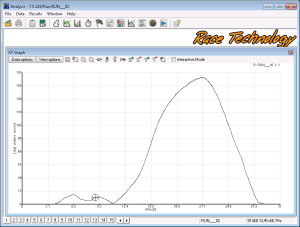The results looked ok. But because there was no RPM signal logged by the datalogger, it isn't possible to calculate the torque the engine was putting out. To do this, some postprocessing of the data was required. So we exported the data to a csv file and got gawk to do the work.

Basically you take the recorded speed vs rpm in 3rd gear, read the speed from the csv file and calculate the rpm. Reading the power output from the engine and using the calculated rpm we're now able to calculate the torque:

torque = ((power / 1.36) * 1000) / ( 2 * 3.14 * (rpm / 60))

Using the calculated information, we write out a new csv file with the missing fields filled in. Then we load the data into a bit of software I wrote to create dynographs. The result looks like this: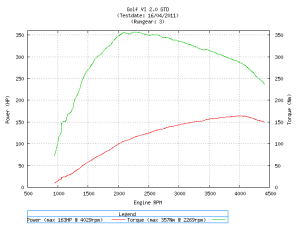The graph looks pretty ok and what you would expect from a turbo diesel.

The interesting bit is going to be that this engine is going to be tuned soon, so we can do a compare measurement and see what the difference is.